• No products in the cart.

# 203.5.14 Neural Network Appendix

In previous section, we studied about Neural Networks Conclusion

In this post we will discuss the math behind a few steps of Neural Network algorithms.

#### Math- How to update the weights?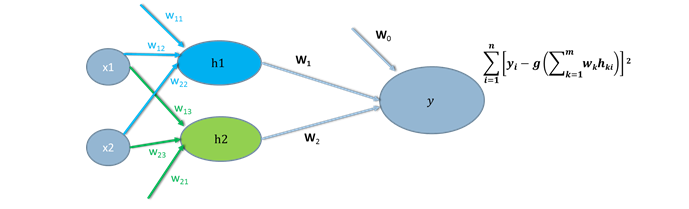• We update the weights backwards by iteratively calculating the error.
• The formula for weights updating is done using gradient descent method or delta rule also known as Widrow-Hoff rule.
• First we calculate the weight corrections for the output layer then we take care of hidden layers.
• $$W_(jk) = W_(jk) + \Delta W_(jk)$$
• where $$\Delta W_(jk) = \eta . y_j \delta_k$$
• $$\eta$$ is the learning parameter
• $$\delta_k = y_k (1- y_k) * Err$$ (for hidden layers $$\delta_k = y_k (1- y_k) * w_j * Err )$$
• Err = Expected output-Actual output
• The weight corrections is calculated based on the error function.
• The new weights are chosen in such way that the final error in that network is minimized.

#### Math-How does the delta rule work?

• Let’s consider a simple example to understand the weight updating using the delta rule.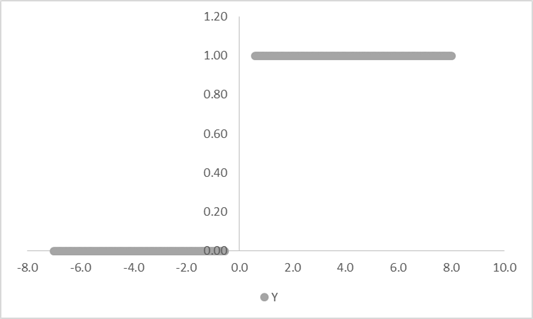• If we are building a simple logistic regression line. We would like to find the weights using weight update rule.
• $$Y= \frac{1}{(1+e^(-wx))}$$ is the equation.
• We are searching for the optimal w for our data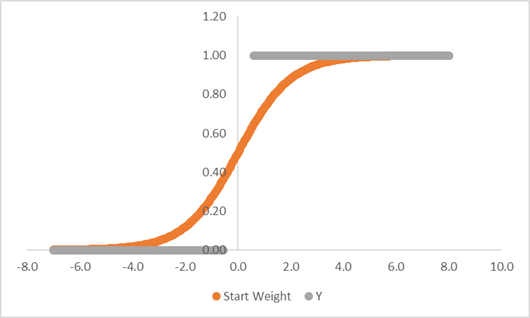• Let w be 1
• $$Y=\frac{1}{(1+e^(-x))}$$ is the initial equation
• The error in our initial step is 3.59
• To reduce the error we will add a delta to w and make it 1.5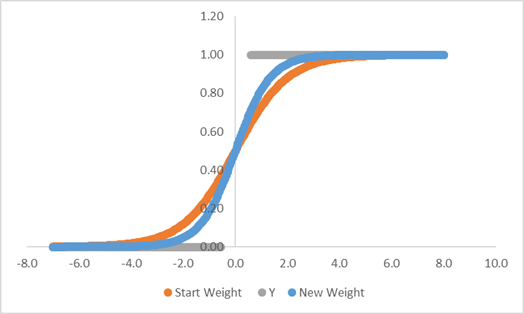• Now w is 1.5 (blue line)
• $$Y=\frac{1}{(1+e^(-1.5x))}$$ the updated equation
• With the updated weight, the error is 1.57
• We can further reduce the error by increasing w by delta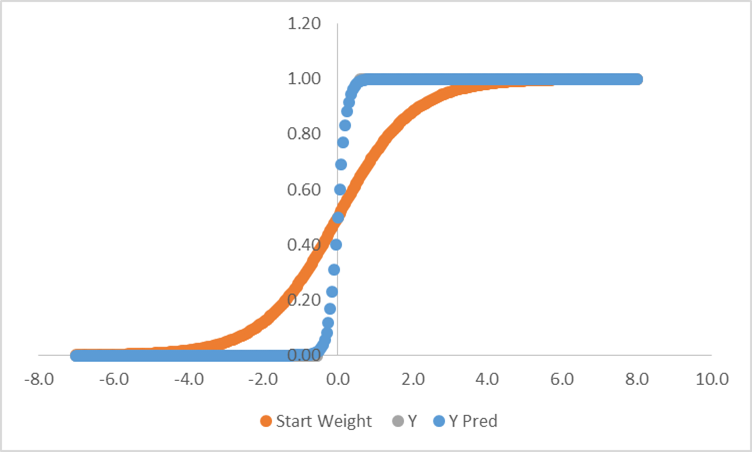• If we repeat the same process of adding delta and updating weights, we can finally end up with minimum error.
• The weight at that final step is the optimal weight.
• In this example the weight is 8, and the error is 0.
• $$Y=\frac{1}{(1+e^(-8x))}$$ is the final equation.
• In this example, we manually changed the weights to reduce the error. This is just for intuition, manual updating is not feasible for complex optimization problems.
• In gradient descent is a scientific optimization method. We update the weights by calculating gradient of the function.

### How does gradient descent work?

• Gradient descent is one of the famous ways to calculate the local minimum.
• By Changing the weights we are moving towards the minimum value of the error function. The weights are changed by taking steps in the negative direction of the function gradient(derivative).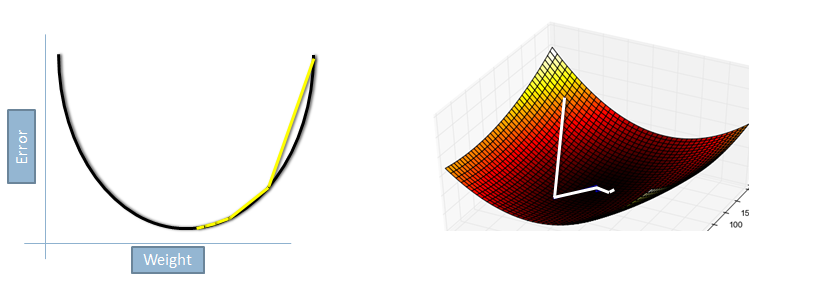#### Does this method really work?

• We changed the weights did it reduce the overall error?
• Lets calculate the error with new weights and see the change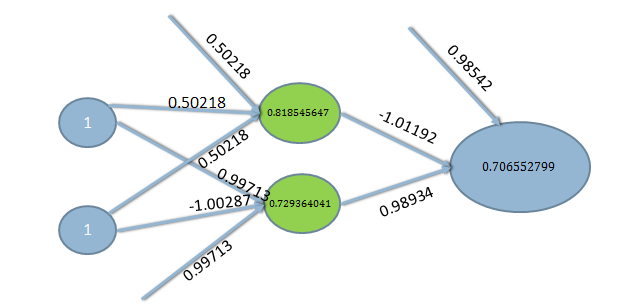• With our initial set of weights the overall error was 0.7137,Y Actual is 0, Y Predicted is 0.7137 error =0.7137.
• The new weights give us a predicted value of 0.70655.
• In one iteration, we reduced the error from 0.7137 to 0.70655.
• The error is reduced by 1%. Repeat the same process with multiple epochs and training examples, we can reduce the error further.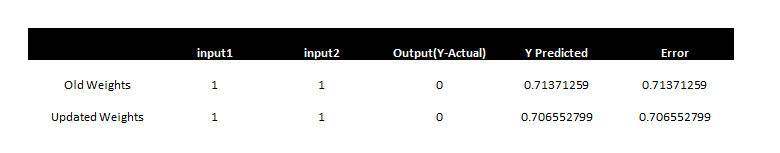### References & Image Sources

In next section, we will be studying about Introduction to SVM

6th November 2017
0 responses on "203.5.14 Neural Network Appendix"